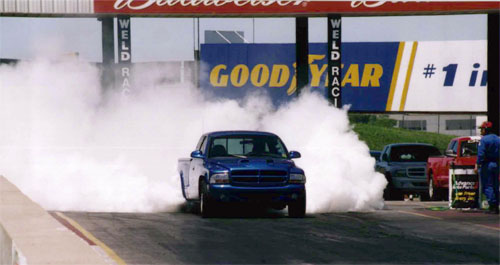# Dodge Dakota R/T Information

###### 2000 Dodge Dakota R/T Information:Above: 2000 Dodge Dakota R/T by Ryan Williams. Featured February 2005 on the Mopars Of The month site. Click Here to check it out.

For 2000 two new colors were added to The Dakota R/T model “Bright White” and “Amber Fire”. Solar Yellow was dropped, but a few were built early in the year. Deep Amethyst was also dropped. The 2000 Dakota R/T comes equipped with a 5.9-liter Magnum V-8 and large-bore, free-flow exhaust that delivers increased engine performance and an exhaust note that is backed up by its performance capabilities.

A four-speed automatic transmission connects the 245 horsepower and 345 lb.-ft. of torque from the 5.9-liter Magnum V-8 to the limited-slip rear axle which turns massive 255/55×17-inch tires. The Dakota R/T’s suspension tuning also includes front and rear stabilizer bars for added control. It’s lowered one full inch and offered in only two-wheel drive configuration. Dodge was expected to produce 5000 units for 2000 but only less than 4,000 were built.

###### 2000 Dodge Dakota R/T Production Numbers

Regular Cab Color:

• Amber Fire: (USA) = 47 – (Canada) = 13 – (Export) = 2 – Total = 62
• Black: (USA) = 316 – (Canada) = 31 – (Export) = 2 – Total = 349
• Bright White: (USA) = 116 – (Canada) = 7- (Export) = 1 – Total = 124
• Flame Red: (USA) = 223 – (Canada) = 14 – (Export) = 3 – Total = 240
• Intense Blue: (USA) = 136 – (Canada) = 22 – (Export) = 4 – Total = 162
• Solar Yellow: (USA) = 25 – (Canada) = 0 – (Export) = 0 – Total = 25

Total 2000 Dakota R/T Regular Cab Production = 962

Club Cab Color:

• Amber Fire: (USA) = 122 – (Canada) = 40 – (Export) = 1 – Total = 163
• Black: (USA) = 1,162 – (Canada) = 170 – (Export) = 2 – Total = 1,334
• Bright White: (USA) = 548 – (Canada) = 37- (Export) = 1 – Total = 586
• Flame Red: (USA) = 802 – (Canada) = 98 – (Export) = 9 – Total = 909
• Intense Blue: (USA) = 534 – (Canada) = 97 – (Export) = 2 – Total = 633
• Solar Yellow: (USA) = 69 – (Canada) = 1 – (Export) = 0 – Total = 70

Total 2000 Dakota R/T Club Cab Production = 3,695